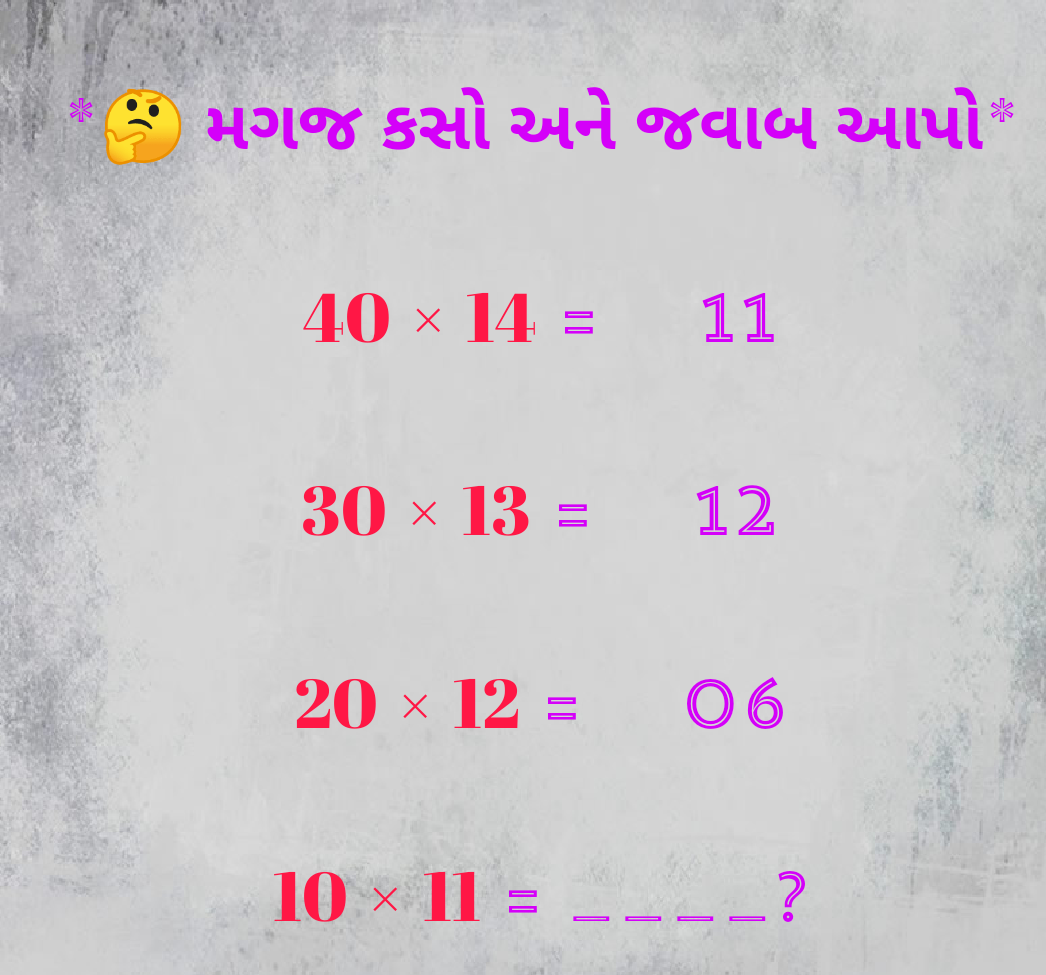## Pages

### MATHS PUZZLE NO-3 WITH ANSWER

Brain Math Puzzle is a game to improver your Logical Skills & Reasoning.

Brain Math Puzzle is a game to improver your Logical Skills & If you like mathematics than this game is made for you.

More than 150 levels in game.

More than 20 different Objects used in a game.

Math puzzle

Simple math puzzle game.

More than 200 different levels.

Take a block from panel and make equation.

Use hints when you getting stuck.

Easy to play hard to master.

How to Play ?

Solve the equation & Give the answer.

For Each game start you will get 3 life.So if you wrong some where you will get another chance.

No time limits.

Who can play ?

Every math lover can play this game no age limit.If u r genius solve it:-

40 × 14 = 11

30 × 13 = 12

20 × 12 = 6

10 × 11 = ?

Logic :

40×14 = 560 = 5+6+0 = 11

30×13 = 390 = 3+9+0 = 12

20×12 = 240 = 2+4+0 = 6

So 10×11 =110 = 1+1+0 = 2

Game Features

•Realistic graphics and ambient sound.

•Realistic 2D stunning and amazing animations.

•Smooth and simple controls.

•User friendly interface and interactive graphics.

Important Point

Use BODMAS Rules & keep Focus on Objects.

Benefits

improves mathematics skills.

Improves logical skill.

Improve reasoning.For Best View Please Open This Website In CHROME / OPERA Browser